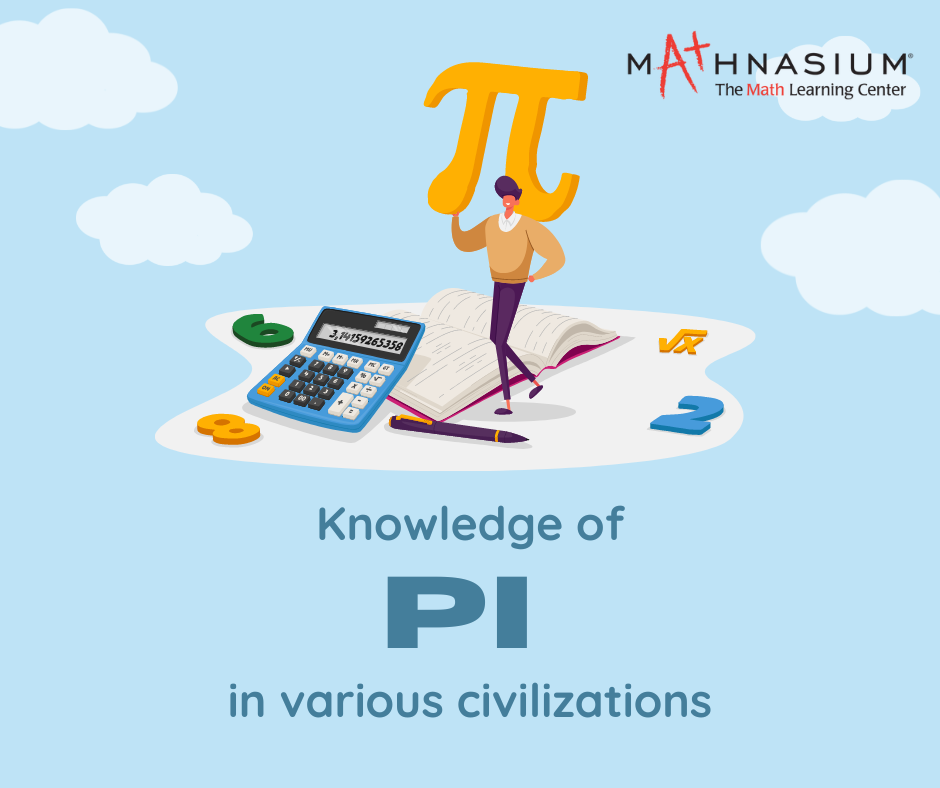877-601-6284
Get Started by Finding a Local Center

# The Unbelievable History of Pi

Mar 7, 2023 | Location Cherry HillMathematical calculations rely heavily on Pi, which remains constant. Pi is used in calculations of area, volume, and other stems of math as well. So, there is no doubt that the value of Pi is of great importance for mathematical studies. The Pi value we have today is the result of ancient civilizations' efforts to determine the correct value of this constant. The history of Pi in Math states that there were countless civilizations with the knowledge to decide the value of Pi. Let us look at some of these civilizations and the values they decided for Pi.

Babylonian : By the 17th century BC, the Babylonians had relatively advanced knowledge of math. They were memorized into complicated tables that expressed squares, fractions, square roots, cube roots, reciprocal pairs, and linear and quadratic equations. It should come as no surprise, then, that these math wizards had also discerned an estimate of pie. According to Babylonians the value of pie was 3 1/8 = 3.125.

Egyptians : The Egyptians also knew pie. The oldest evidence of pie is found in the Rhind Papyrus, which dates from about 1650 BC. The Egyptian calculated the value of pie as 4 × (8/9)² = 3.16

Greek : The Greeks significantly advanced the study of mathematics, particularly the field of geometry. In the 3rd century BC, Archimedes, the great engineer, and inventor devised the first known theoretical calculation of pie. According to Archimedes the value of pie was 223/71 < π < 22/7. At this point, Archimedes's calculation was around 3.1418, which was by far the closest approximation up to this point. About 400 years later, another Greek, Ptolemy, further refined the estimate of the pie using the chords of a circle with a 360-sided polygon to obtain π = 3 17/120 = 3.14166.

Chinese : The Chinese mathematician, Zu Chongzhi, calculated the value of a pie to six decimal places and demonstrated the value of a pie as 3.1415926 < π < 3.1415927. Mathematicians began using the Greek letter π in the 1700s. Introduced by William Jones in 1706, the use of the symbol was popularized by Leonhard Euler, who adopted it in 1737.

Knowledge of pi in ancient civilizations contributed to today's accurate value without a shadow of a doubt. From the Middle Ages to the Modern Era, further developments have been made to calculate Pi's exact value.

##### Locations near
Could not find Center, try again